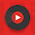## Objective Questions From Basic Electrical Engineering:

 Which of the following can vary with ac, but never with dc?
A.  Power
B.  Voltage
C.  Frequency
D.  Amplitude

 If all other factors are kept constant, the inductance of the air-core coil is not affected by
A.  The frequency
B.  The number of turns
C.  The diameter of the coil
D.  The length of the coil

 The length of time between a point in one cycle and the same point in the next cycle of an ac wave is the
A.  Frequency
B.  Magnitude
C.  Period
D.  Polarity

 Capacitance acts to store electrical energy as
A.  Current
B.  Voltage
C.  A magnetic field
D.  An electric field
 On a spectrum analyzer, a  ac signal having only one frequency component looks like
A.  A single pip
B.  A sine wave
C.  A square wave
D.  A sawtooth wave

 A ferromagnetic core is place in the inductor to
A.  Increase the current carrying capacity
B.  Increase the inductance
C.  Limit the current
D.  Reduce the inductance

 The period of a ac wave, in seconds is
A.  The same as the frequency in hertz
B.  Not related to the frequency in any way
C.  Equal to 1 divided by the frequency in hertz
D.  Equal to the peak amplitude in volts divided by the frequency in hertz

 An inductor works by
A.  Charging a piece of wire
B.  Storing energy as a magnetic field
C.  Choking off dc
D.  Introducing resistance into a field

 As capacitor plate area increases
A.  The capacitance increases
B.  The capacitance decreases
C.  The capacitance does not change
D.  The current handling ability decreases
 In a small inductance
A.  Energy is stored and released slowly
B.  The current flow is always large
C.  The current flow is always slow
D.  Energy is stored and released quickly

7:49 PM

#### 1 comment:

1.NICE COLLECTIONS